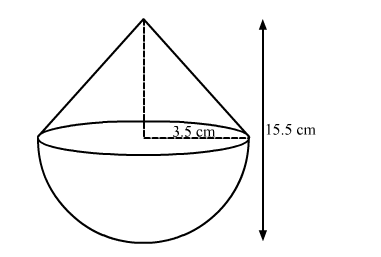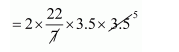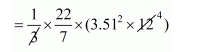# A toy is in the form of a cone mounted on a hemisphereQuestion:

A toy is in the form of a cone mounted on a hemisphere of radius 3.5 cm. The total height of the toy is 15.5 cm find the total surface area and volume of the toy.

Solution:Radius of hemisphere = 3.5 cm

Total height of the toy = 15.5 cm.

Surface area of cone

$=\pi r l$

$I=\sqrt{(12)^{2}+(3.5)^{2}}$

$=\sqrt{156.25}$

$=12.5 \mathrm{~cm}$

Therefore,

Surface area of cone

$=\frac{22}{7} \times 3.5 \times 12.5$

$=137.5 \mathrm{~cm}^{2}$

Surface area of hemisphere

$=2 \pi r^{2}$$=77 \mathrm{~cm}^{2}$

Therefore,

Total surface area of the toy

$=137.5+77$

$=214.5 \mathrm{~cm}^{2}$'

Volume of cone

$=\frac{1}{3} \pi r^{2} h$$=154 \mathrm{~cm}^{2}$

Volume of hemisphere

$=\frac{2}{3} \pi r^{3}$

$=\frac{2}{3} \times \frac{22}{7} \times(3.5)^{3}$

$=89.83 \mathrm{~cm}^{3}$

Therefore,

Total volume of the toy

$=(154+89.83) \mathrm{cm}^{3}$

$=243.83 \mathrm{~cm}^{3}$